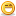# Thread: I'm a bit confused, help

1. ##I'm a bit confused, help

http://science.slashdot.org/science...7/0416223.shtml

Fun story, lame math.

I did a problem like this 10 odd years ago. Maybe some of you math nerds can give me an insight on this.

(i tried to be concise but it's still a bit long)

Most mathmetians say that infinity is not a real value and that therefore we cannot use it it mathematical formulations because it is an abstraction rather than a real number. The same people say that 1/0 cannot = infinity because a) division by zero is not a real operation due to it's possibility
b) nothing can equal infinity because infinity is an abstraction and cannot be used in mathematical formulations.
Just because two things aren't real and seem to express the same value, it doesn't mean they equal the same value. ∞ != 1/0.

Why the panic? Because of one.

9 * 1 = 9.

8 * 1 = 8.

x * one = x. Why? Because multiplication is a set oriented function. 1 set (or carton) of 12 eggs = 12. 2 sets of 12 eggs = 24. 1/2 a carton = 6 eggs.

But we know that any x / x = one. 12 eggs / 12 eggs per carton = 1 carton.

So if assumption 1 is true (x * 1 = x) and assumption 2 is true (x / x = 1) than assumption 3 is true (x1 * (x2 / x2) = x1) .

And if that is true and ∞ = 1/0 (the equal sign is a pandora's box) then the product of ∞ and 0 (∞/∞ or 0/0) = 1.

9 * 0/0 = 8* 0/0 = 0/0. Divide out one and you get 9=8.

Anyways, that's the fear. Unreal abstractions used in real math breaks the assumptions of real math down. And it's not like people have to divide things by zero in real life anyways. I mean lets look at physics for instance. Newton wrote equations for things that move and he didn't need infinity.

Velocity = distance / time. time = distance / velocity. You run at 10 m/s. Your course is 10 meters. You'll cross the finish line in t = 10m / 10m/s = 1 second.

But Newton is old. Physics is updated. Time has a brief history in the world of Stephen Hawking. Speaking of Steven, he runs at 0 m/s. Lets give him a course of 50 cm. He'll cross the finish line in... well it won't be infinity seconds, no sir. This scenario cannot exist because it violates the rules of real math. Divison by zero is a dangerous concept to many modern pythagorians.

I think we can adapt infinity and zero into real math constructs but we have to change our assumptions a bit.Reply With Quote

2. ##if 1 is divided by nothing (0) it remains 1. Similarly if 1 is multiplied by nothing, it also remains 1. What's am I missing here...?Reply With Quote

3. ##The /0 is the problem

Compare:

I have no pizza and three people; how much pizza does each person get? That question makes sense p=0/3=0

I have 3 pizzas and no people; how much pizza does each person get? That question makes no sense and can't be answered.

Any time we have to divide by 0 the question becomes invalid: we say undefined rather than ascribing an infinity to the answer. Some say there are many kinds on infinity but that's a bit philosophically difficult. Infinity is not a actually number and no mathematical operations can be done on it.

I have insomnia....

I think you are right. Check out Gabriel's horn if you want more brain-burn or Benford's law (that's a real head-scratcher)For a real challenge: try to work out what is going on in UK politics. I can't make any sense of it.Reply With Quote

####Posting Permissions

• You may not post new threads
• You may not post replies
• You may not post attachments
• You may not edit your posts
•